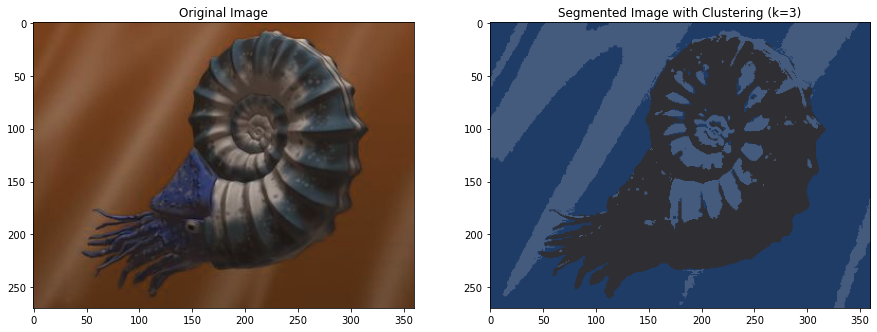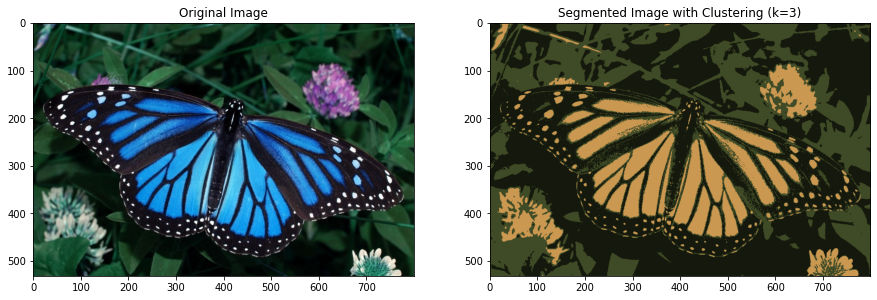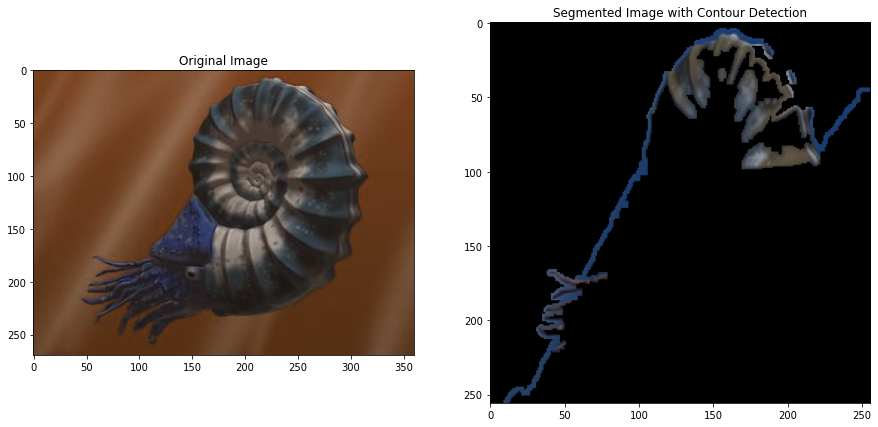Betul Mescioglu

# Image Segmentation:

Creating segments by grouping pixel values of an image using smart masks is known as Image Segmentation. It helps in reducing the complexity of the image to make further processing or analysis of the image simpler. There are two approaches in image segmentation; similarity and discontinuity. Similarity approach uses a threshold to detect the similarities between pixels to separate surfaces. Clustering uses this approach. Discontinuity approach detects discontinuity of pixel intensity values of the image. Line, point and edge detection techniques use this approach.
In this project we will use K-means, Contour detection and Thresholding methods to segment images.

### Segmentation using K-Means Clustering:

K-means clustering is an unsupervised learning method where similar pixels are assigned to the same clusters. The essence of k-means is, we form cluster centers first (in this case three cluster centers), then, as we obtain new pixel values from the image, they are assigned to either one of the clusters based on how close their intensity value is to the center of clusters. We update the centroids of the clusters and continue this process until all pixels are exhausted. In the following, we group pixels in the images into three clusters (k=3).

``````#Image Segmentation using K-means

##Importing libraries and Images

import matplotlib.pyplot as plt
import numpy as np
import cv2``````
``````path1 = 'spiral.jpg'
path2 = 'butterfly.jpg'
images=[img1, img2]``````
``````for i in images:
##Preprocessing the Image
img = cv2.cvtColor(i,cv2.COLOR_BGR2RGB)
twoDimage = img.reshape((-1,3))
twoDimage = np.float32(twoDimage)

##Defining Parameters
criteria = (cv2.TERM_CRITERIA_EPS + cv2.TERM_CRITERIA_MAX_ITER, 10, 1.0)
K = 3
attempts=10

##Apply K-Means
ret,label,center=cv2.kmeans(twoDimage,K,None,criteria,attempts,cv2.KMEANS_PP_CENTERS)
center = np.uint8(center)
res = center[label.flatten()]
result_image = res.reshape((img.shape))
fig, ax = plt.subplots(1,2, figsize=(15,10))
ax=ax.ravel()
ax.imshow(i)
ax.set_title("Original Image")
ax.imshow(result_image)
ax.set_title("Segmented Image with Clustering (k=3)")
plt.show()
``````### Analysis:

We see that, in both cases, the variety of colors in the original images are restricted to three colors in the segmented images, as we grouped them into three clusters. If we had more clusters, the segmented images would be closer to the original images, as more variety of colors would be introduced.

### Segmentation using Contour Detection:

Image segmentation using contour detection can be achieved in several steps:

• Find the average pixel values and use this as the threshold to be used by Canny edge detection.
• Apply dilation to the edges found by Canny edge detection.
• Create contours of the detected edges.
• Create an empty mask and draw the contours.
• Apply this mask on the original image to black out irrelevant parts.
``````for i in images:
#Image Segmentation using Contour Detection
img = cv2.resize(i,(256,256))

gray = cv2.cvtColor(img,cv2.COLOR_RGB2GRAY)
_,thresh = cv2.threshold(gray, np.mean(gray), 255, cv2.THRESH_BINARY_INV)
edges = cv2.dilate(cv2.Canny(thresh,0,255),None)

##Detecting and Drawing Contours

cnt = sorted(cv2.findContours(edges, cv2.RETR_LIST, cv2.CHAIN_APPROX_SIMPLE)[-2], key=cv2.contourArea)[-1]

##Segmenting the Regions